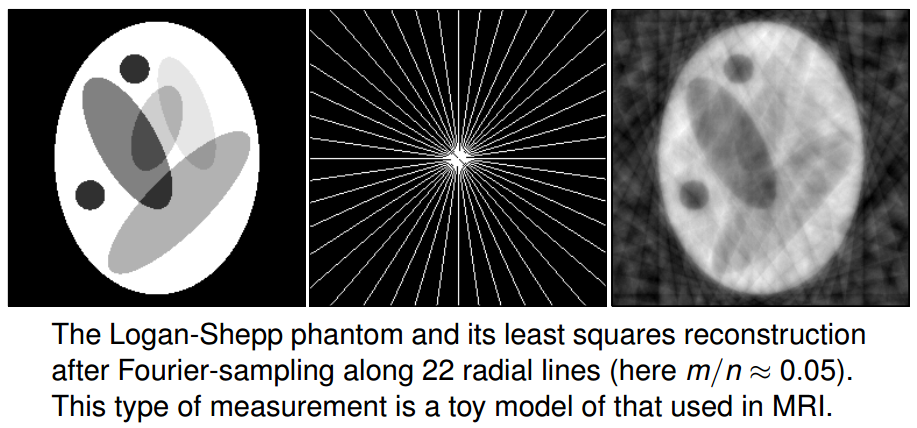# 压缩感知（一）x$x$满足一定的稀疏性时，就能够获得一定的唯一解。其中s-稀疏向量的定义如下

s:={xN:||x||0s}

## 原理

RnRNΔ$R^n到R^N的映射记为\Delta$，则还原效果可以表示为||x0Δ(Θx0)||X$||x_0-\Delta (\Theta x_0)||_X$，其中X$X$表示一定范数。当x0$x_0$中非0元素较少时，一种自然的解码即是如下规划问题的解

P0minxN||x||0s.t.Θx=y

Δ(Θx0)=x0

P1minxN||x||1s.t.Θx=y

P2mintNt1+t2+...+tNs.t.Θx=yti0,i=1,2,...,N.tjxjtj

||vT||1||vTc||1,T{1,...,N},#T=s.

Δ(Θx0)=x0

(1σs)||x||2||Θx||2(1σs)||x||2

Δ(Θx0)=x0

||Δ(Θx0)x0||2C0σs(x)1s,C0σs(x)X=minzs||xz||XxNσs(K)X=minxkσs(x)XKN

Δ(Θx0)=x0x2s,

nc1slog(Nc2s),c1=1log9c2=4